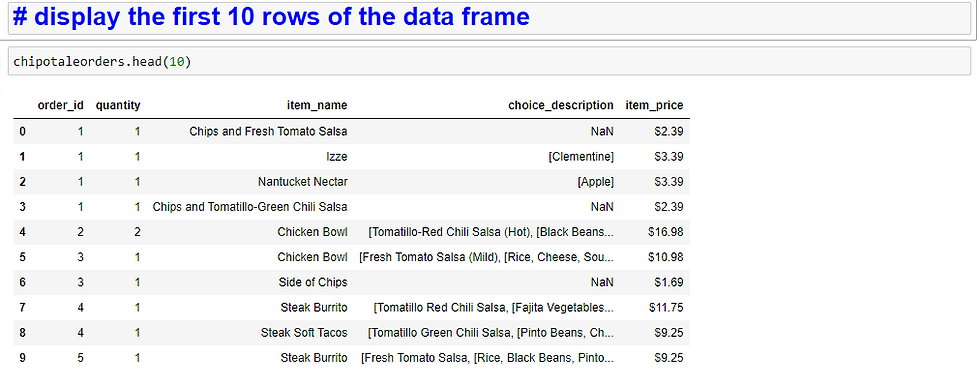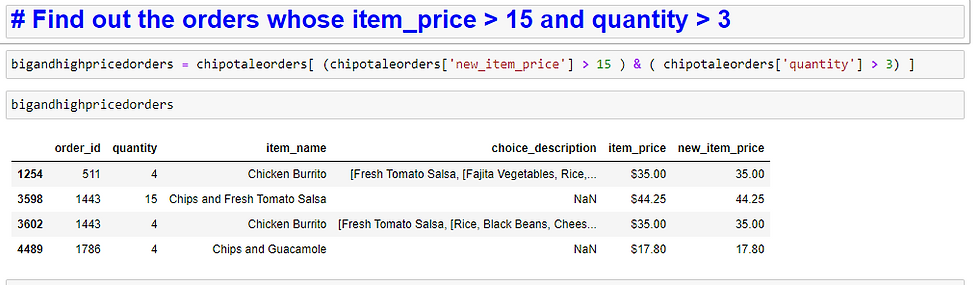top of pageSearch

# Exploratory Data Analysis (EDA) using Pandas and Matplotlib library in Python.## What is Pandas?

Pandas is a data analysis module for the Python programming language. It is open-source and BSD-licensed. Pandas is an add-on software library created by Wes McKinney for the Python programming language.

1. Pandas library is a very powerful tool to convert data from CSV format to data frame which is basically rows and columns.

2. Pandas library has functions like shape, describe(), dtype() that can be used to inspect the data and perform broader analysis like how many rows and columns are present, what is the data type of each column, are there any missing values?

3. Let’s learn how to use panda library in co-ordination with matplotlib, to display bar graph.## What is Matplotlib?

Matplotlib is a comprehensive library for creating static, animated, and interactive visualizations in Python. Matplotlib makes easy things easy and hard things possible.

## Why data visualization and plots are important?

A plot is a graphical technique for representing a data set, usually as a graph showing the relationship between two or more variables. The plot can be drawn by hand or by a computer. In the past, sometimes mechanical or electronic plotters were used.

Before conducting a meaningful investigation, it’s important to organize the data you collected. By organizing data, a scientist can more easily interpret what has been observed.

Organizing data comprises of steps such as

1. Remove duplicate records.

2. impute missing values.

3. Normalize data.

Since most of the data scientist collect is quantitative data. Tables and charts are usually used to organize this information. Graphs are created from data tables. They allow the investigator to get a visual image of the observations, which simplifies interpretation and drawing conclusions. Valid conclusions depend on organization and clear interpretation of data.

## What is Seaborn?

Seaborn is "another" visualization library. It builds on Matplotlib foundations but renders more sophisticated graphs. Seaborn makes it easy to generate certain kinds of plots such as heat maps, time series and violin plots, box plots.

## EDA Implementation steps (in jupyter notebook)

Install Pandas

The Pandas module isn’t bundled with Python, so you can manually install the module with pip.

`pip install pandas`

import Pandas and matplotlib

```import pandas as pd
import matplotlib.pyplot as plt```

Read a tabular data file using pandas

Data set information: Lets analyse the data from Chipotle, a popular Mexican fast-food chain in north America.Read and display first 10 rows.Identify the shape of the Data frame.It tells us there are 4622 rows and 5 columns in a data frame.

Find and display Column names.Find data types of the columnsFind out the orders of quantities are greater than 3.Find out the high-priced orders (with item price greater than \$15?)How do I apply multiple filter criteria to a pandas DataFrame?After analyzing tabular data in the above example, now lets analyse data from CSV format.

Tokyo Olympic data set from KaggleRead and display first 10 rows.How to find the statistical information about the numeric columns present in data frame?From above table, observations from the bottom row, we can see the maximum gold medals earned are 39. Maximum silver medals earned are 41, and Total medals earned are 113.

How to apply multiple filter criteria to a pandas Data Frame?How to sort a pandas DataFrame or a Series? Let's sort the data frame by “Rank by Total” ascending order and fetching first 20 countries.Plot the graph for the above using matplotlibChoose the different color palate for the graph.Use the query to filter data frame.Plot the bar graph showing gold medals won by each country.## Conclusion

1. Pandas is a fast, powerful, flexible and easy to use open-source data analysis and manipulation tool, built on top of the Python programming language.

2. Matplotlib is an amazing python library that can be used to plot Pandas data frame.

## References

pandas documentation — pandas 2.0.3 documentation (pydata.org)

Tokyo 2020 Olympics Medals | Kaggle

Learn Python Programming — Python Tutorial (pythonbasics.org)